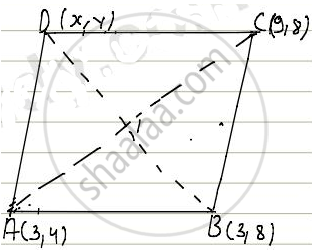Share

# The Three Vertices of a Parallelogram Are (3, 4) (3, 8) and (9, 8). Find the Fourth Vertex. - CBSE Class 10 - Mathematics

ConceptConcepts of Coordinate Geometry

#### Question

The three vertices of a parallelogram are (3, 4) (3, 8) and (9, 8). Find the fourth vertex.

#### Solution

Let A(3,4), B(3,8) and C(9,8) be the three given vertex then the fourth vertex D(x,y)Since ABCD is a parallelogram, the diagonals bisect each other.

Therefore the mid-point of diagonals of the parallelogram coincide.

Let p(x,y) be the mid-point of diagonals AC then,

P(x,y) = ((3 + 9)/2, (4 + 8)/2)

P(x,y) = (6,6)

Let Q(x,y) be the mid point of diagonal BD. then

Q(x,y) = ((3 + x)/2, (8 + y)/2)

Coordinates of mid-point AC = Coordinates of mid-point BD

P(x,y) = Q(x,y)

=> (6,6) = ((3 + x)/2, (8 + y)/2)

Now equating individual components

=> 6 = (3 + x)/2 and 6 = (8 + 4)/2

=> 3 + x = 12 and 8 + y = 12

=> x = 9 and y = 4

Hence, coordinates of fourth points are (9, 4)

Is there an error in this question or solution?

#### Video TutorialsVIEW ALL 

Solution The Three Vertices of a Parallelogram Are (3, 4) (3, 8) and (9, 8). Find the Fourth Vertex. Concept: Concepts of Coordinate Geometry.
S